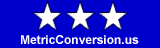Metric System Prefixes

Metric Conversion > Metric System • Metric Prefixes

Table of metric system prefixes, symbols, and multiplication factors.

The metric system defines prefixes and corresponding symbols for positive and negative powers of 10, as applied to each unit of measure.  These prefixes are defined for one power of ten increments in the more middle range and for three powers of ten increments in the outlying ranges.  For instance, the meter is the standard unit of length in the metric system.  The decimeter is one tenth of a meter, the centimeter is one hundredth of a meter, the millimeter is one thousandth of a meter, and the micrometer is one millionth of a meter.  In practice, some prefixes and/or combinations are rarely used.  The kilometer (one thousand meters) is a commonly used measure, while the dekameter (ten meters) and the hectometer (one hundred meters) are rarely used.  The prefixes at the top and bottom of the table are mostly used in specialized mathematical and scientific fields.

This convenient table of metric system prefixes is useful when doing metric conversion calculations.

Metric System Prefix Table
PrefixSymbolMultiplication FactorPower of 10
yotta Y1,000,000,000,000,000,000,000,000+24
zetta Z1,000,000,000,000,000,000,000+21
exa E1,000,000,000,000,000,000+18
peta P1,000,000,000,000,000+15
tera T1,000,000,000,000+12
giga G1,000,000,000+9
mega M1,000,000+6
kilo k1,000+3
hecto h100+2
deka da10+1
deci d0.1-1
centi c0.01-2
milli m0.001-3
micro µ0.000,001-6
nano n0.000,000,001-9
pico p0.000,000,000,001-12
femto f0,000,000,000,000,001-15
atto a0,000,000,000,000,000,001-18
zepto z0,000,000,000,000,000,000,001-21
yocto y0,000,000,000,000,000,000,000,001-24

Other Metric System Resources

• SI Prefixes - discussion and table of metric system SI prefixes.